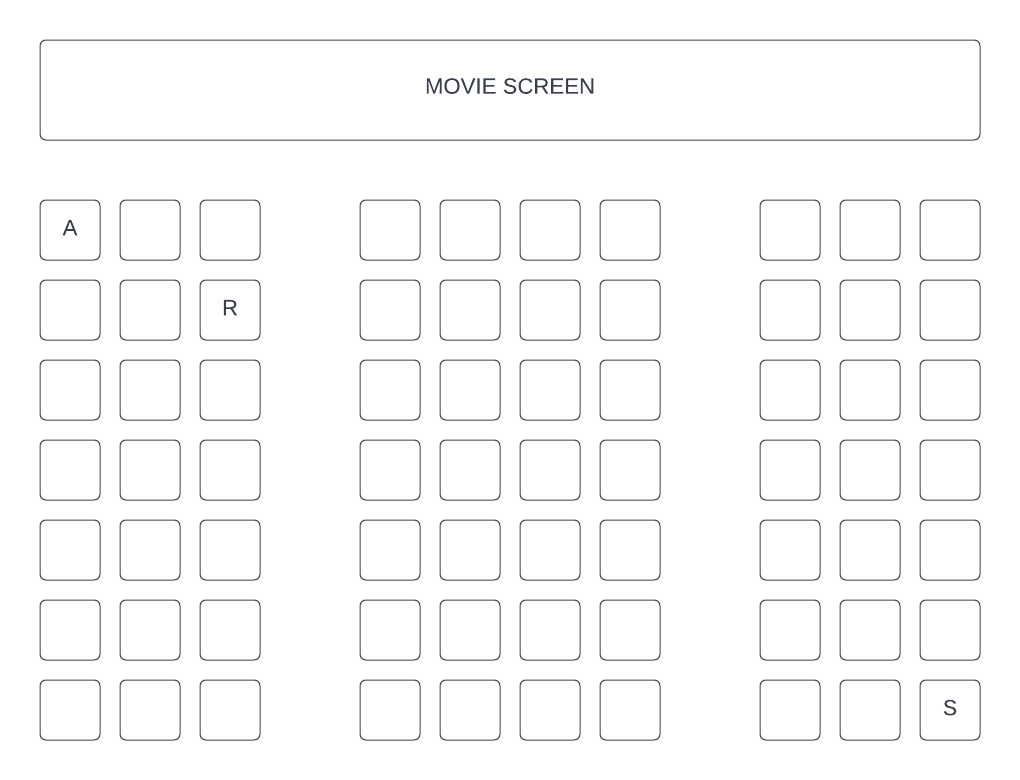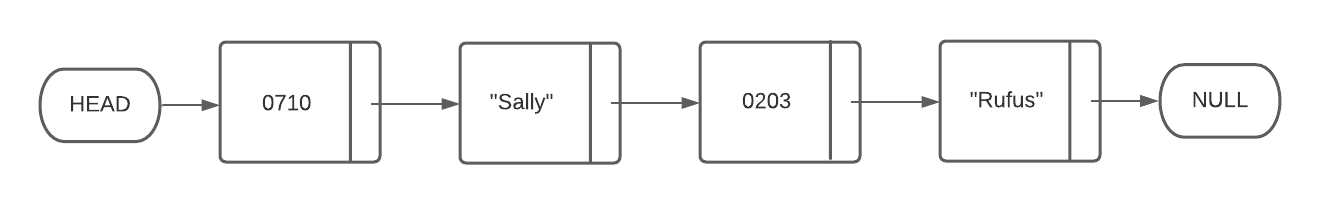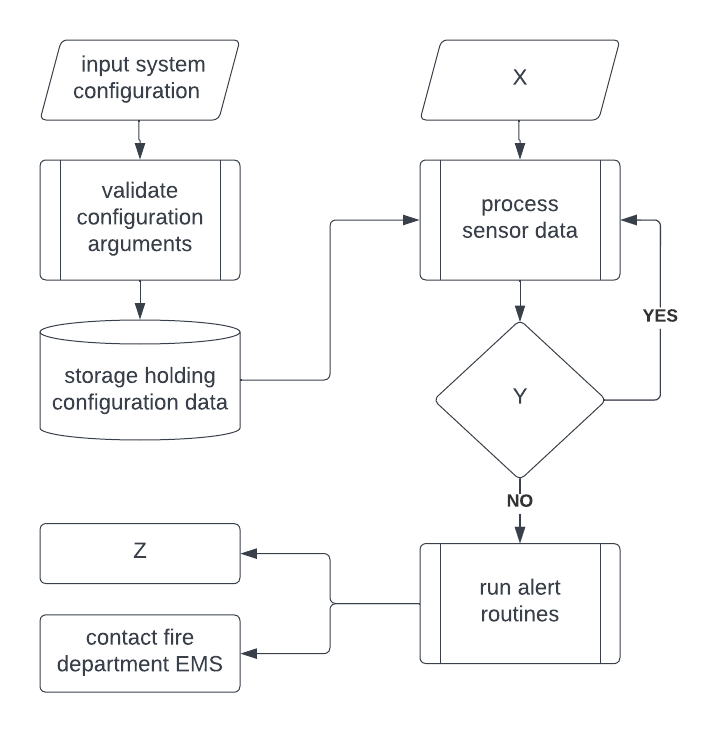Login or Create an Account to view question mark schemes, comment, and download a PDF of this test
Question 1[6 Marks]
Consider the following boolean expression:
(A AND NOT B) OR (B AND NOT A)
(a).
Construct a truth table for the boolean expression above.

(b).
Hence or otherwise, state the simple boolean expression composed of only one logical operator which is logically equivalent to the boolean expression above.

Question 2[14 Marks]
Consider the following diagram representing seats in a movie theater where each seat is specified by a row number ranging from 1 to 7 (inclusive) and a column number ranging from 1 to 10 (inclusive). The theater's ticketing system has already allocated Andrew ("A"), Sally ("S") and Rufus ("R") seats for an upcoming screening.The ticketing system stores ticketing information in a collection called TICKETS. TICKETS is composed of nodes where values alternate between integers and strings - each integer has encoded seating information for the customer whose name is held as a string in the next node. The first two digits and the last two digits of each integer specify the row number and column number of a seat respectively. For example, Rufus has a ticket for the third seat across in the second row. TICKETS contains only those customers with a ticket and all ticket holders have exactly one allocated seat each. In TICKETS, it can be assume that a customer name will always follow their seating number. Therefore, customer seating information is always held in sequentially linked pairs - for example:(a).
Construct an algorithm to only output all ticket holder names held in TICKETS.

(b).
Describe one problem with storing ticketing information in this way and explain why a 2D array would be more appropriate.

(c).
Given a 7x10 2D array called TIX, construct an algorithm to parse ticketing information from the collection TICKETS into TIX such that ticket holder names are copied to a TIX location representative of their seat location. For example, TIX, TIX, and TIX should hold the names "Andrew", "Rufas" and "Sally" respectively. Unassigned seats should be indicated in TIX by the string "Empty".

Question 3[2 Marks]
Consider the following array of Cartesian co-ordinates called POINTS.
POINTS = [(3,7),(5,11),(1,2),(4,9)]
Consider the following pseudocode which draws a path connecting each point contained in the array POINTS.
drawLine((3,7),(5,11)) drawLine((5,11),(1,2)) drawLine((1,2),(4,9))
Rewrite the pseudocode above to achieve the same functionality both iteratively and more generally, such that POINTS may contain any four coordinates, each of the form (a, b).
Question 4[1 Mark]
State the advantage of a Circular Queue over a Linear Queue
Question 5[4 Marks]
Explain how the OSI model helps with abstraction.
Question 6[4 Marks]
Construct both a logic diagram and truth table for the following expression:
NOT A OR A AND B
Question 7[2 Marks]
Define the term heuristics.
Question 8[10 Marks]
A busy cafe has decided to streamline their business by implementing a software assisted ordering system. The new system will record customer orders at the front counter whereupon they are automatically added to a queue of orders accessed by a barista working at the back of the cafe to fulfill orders uninterrupted.
(a).
State one reason why a queue would be better suited to holding coffee orders than a stack.

(b).
The new systems holds the price for each type of coffee in an array called ITEMS as follows:

+--------------+--------------+ | Espresso | 1.20 | | Latte | 2.50 | | Cappuccino | 3.70 | +--------------+--------------+
Coffee orders are held in a queue called ORDERS where each order is a single integer indicating the type of coffee to be made by their respective row index in ITEMS array.
Additionally, a 3x3 2D array called SALES is initially defined as follows:
+--------------+--------------+--------------+ | Espresso | 0 | 0 | | Latte | 0 | 0 | | Cappuccino | 0 | 0 | +--------------+--------------+--------------+
Given ITEMS, ORDERS and SALES, write pseudocode such that column one and column two contain the total number of orders and total income for each coffee type respectively.
For example, the following ORDERS queue:
+-------+-------+-------+-------+-------+ 1 0 1 2 0 +-------+-------+-------+-------+-------+
would result in the following SALES array:
+--------------+--------------+--------------+ | Espresso | 2 | 2.40 | | Latte | 2 | 5.00 | | Cappuccino | 1 | 3.70 | +--------------+--------------+--------------+
(c).
The method rowswap(ARR, ROWA, ROWB) acts on the array ARR provided to swap the row at index ROWA with the row at index ROWB.

Without using loops, write pseudocode to sort the rows of SALES from lowest to highest total income. The row for the coffee with the highest total income should be at row index 2.
Question 9[1 Mark]
Choose the one option which best describes the World Wide Web (WWW).
(a).
A globally interconnected network of networks
(b).
A way of sharing resources via hypertext and hyperlinks
(c).
A way for data to travel via network protocols
(d).
The internet
Question 10[11 Marks]
(a).
Given a short array of integers of length 2 called myShortArray, write pseudocode to determine and output the biggest and second biggest number.

(b).
Given an array of integers of length 5 called myLongerArray, write pseudocode to determine and output the biggest number it contains.

(c).
Given an array of integers of length 7 called myArray, write pseudocode to output the sum of the two biggest integers it contains. The algorithm should handle both negatives values and repeated values.

Question 11[2 Marks]
Given an empty array called NUMBERS of size 100, set each location which has an index that is a multiple of 5 (includes 0) to the number 7 and all remaining elements to the value 3.
Question 12[3 Marks]
Write an algorithm which outputs every item of a 2D array that is 100 by 100 elements in size
Question 13[4 Marks]
Input the dimensions of a rectangle then print both area and perimeter.
Question 14[3 Marks]
Input 3 numbers and if all three numbers are different then output "distinct", otherwise output "not distinct".
Question 15[6 Marks]
The process of detecting a building fire is carried out by ionisation-type smoke detectors / sensors which are continuously monitoring the flow of ions (current) between two electrically charged plates. If the ion flow between the plates is sufficiently disrupted by smoke, alarms are sounded across the building to alert occupants. If the activated alarms are not turned off within the period of time programmed in system configuration, a message is sent via a fast and reliable fibre optic internet connection to the local fire department’s Emergency Management System (EMS).
(a).
Explain relationship between sensors, processor(s) and output transducers in this scenario.(b).
Provide appropriate labels to replace X, Y & Z in order to complete the system flowchart above for the scenario described.

Question 16[1 Mark]
Given an array containing 7 names called NAMES, the following algorithm outputs the first name found starting with “M”. It uses a utility function called firstLetter to return the first letter of a string - for example, firstLetter(“Kate”) will return the letter “K”.
N = 7 FIRST = “” FOUND = false i = 0 loop while i < N VALUE = NAMES[i] if firstLetter(VALUE) = "M" AND FOUND = false then FIRST = VALUE FOUND = true end if i = i + 1 end loop output FIRST
While the above algorithm functions as intended, suggest a suitable pseudocode modification to improve efficiency.
Question 17[5 Marks]
Describe a recursive function called reverse(buffin, buffout, hay) which moves values from a queue called buffin into another queue called buffout. Use an intermediate stack called hay such that resultant numbers in buffout are, with respect to their original sequence in BUFFIN, in reverse order. Both buffout and hay are initially empty.
Question 18[4 Marks]
Input 7 numbers and use a loop to output their total.
Question 19[1 Mark]
Determine the hexadecimal value of the following binary expression 10 1011 1010 1101
Question 20[4 Marks]
Explain the relationship between exploration and mutation rate in Genetic Algorithms (GAs) and how, in the case of the Travelling Salesman Problem (TSP) scenario presented, the mutation rate affects a potential solution for the shortest tour of the 20 cities.
Question 21[4 Marks]
Outline one advantage and one disadvantage of Tournament Selection when compared to Truncation Selection.
Question 22[1 Mark]
Given a simple traffic light with a pedestrian crossing button forms an open loop system, outline one way in which traffic light operations may return to normal following a pedestrian crossing event.
Question 23[3 Marks]
In an effort to better understand city traffic flows, traffic lights were upgraded to include CCTV cameras.
Discuss the social implications of monitoring traffic using CCTV.
Question 24[7 Marks]
The Medicare Levy Surcharge (MLS) is a 1-2% levy paid by Australian taxpayers who do not have private hospital cover and who earn above a certain income. The current income threshold is AUD\$90,000 for singles and AUD\$180,000 for couples / families (2020-2021 financial year). Once Australians earn above these thresholds within a financial year, they must pay the MLS. Given an annual income called INCOME, a boolean flag for having private hospital cover or not called PRIVATE and another boolean flag for being single or not called SINGLE, write pseudocode to output True if eligible for the MLS or False otherwise. For example, a single (SINGLE = true) person who doesn’t have private health insurance (PRIVATE = false) and is earning AUD\$120,000 (INCOME = 120000) annually must pay the MLS (output True)
Question 25[10 Marks]
(a).
Determine the decimal value of the binary ‘1001’

(b).
Determine the binary value of the denary ‘67’

(c).
Outline why the binary number system is used in computing

To convert decimal to binary, we use a simple handwritten algorithm, this same logic can be applied in pseudocode.
(d).
In pseudocode, using a stack, write an algorithm that finds the binary value of a decimal input by outputting the digits one by one


HomeThe Right Test - Fast